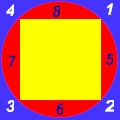High School MathLet's call the radius of the circle R.
The area of the blue square is (2R)^2 = 4R^2
The area of circle is Pi*R^2
The diagonal of the yellow square is 2R, making each side Sqrt(2)*R. The area of the yellow square is 2R^2

The area of  (1+2+3+4) = Blue Square Area - Circle Area = 4R^2 - Pi*R^2 = (4-Pi)*R^2 = 0.86*R^2
The area of (5+6+7+8) = Circle Area - Yellow Square Area = Pi*R^2 - 2R^2 = (Pi-2)*R^2 = 1.14*R^2

We can see that the red pieces are always bigger than the blue pieces.  Therefore, area5 > area1